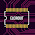# 500W-2000W Power Amplifier SOCL 504

The SOCL 504 Power Amplifier circuit is now possible again as the trend amplifier, is simple and the quality is good for indoor and outdoor sound and cheap power amplifier circuit. Well, turn your trying to listen to how the 500-Watt SOCL that in fact, the result of the development of 150 Watt OCL modified for high voltage above 40VDC.

Power Amplifier SOCL 504 500 Watt has tested with 45V DC and 80V DC voltage is still good and flat sound almost all the frequency obtained and still possible in the modification to tef to get bass thicker and an addition of the number of final transistors to increase the power of 18-inch speakers.

Three transistors are VAS deliberately bolted on the PCB board though not hot, just the model let it be different from the original version. To get the maximum output power the power amplifier circuit requires a DC voltage source with a large current so that the power supply for this power amplifier circuit should use minimal transformer 20 A can be given at least 45V DC to 80V DC.

Below the circuit diagram of power amplifier SOCL 504:

Parts Power Amplifier:
Resistor
R1= 100K, R2=33K, R3=560R, R4=27K, R5=56R, R6=1K, R7=27K, R8=27K, R9=56R, R10=560R, R11=4K7, R12=10K, R13=330R/2W, R14=330R/2W, R15=0.22-0.5R/5W, R16=0.22R-0.5R/5W, VR1=100R-1K

Capacitor
C1=22N, C2=1N, C3=22uF/50V, C4=22uF/100V, C5=22uF/100V, C6=100pF, C7=100pF, C8=100pF, C9=100N, C10=100N C11=22uF/100V

Diode
D1-D5=1N4002

Transistor
Q1-Q3=2N5401, Q4=MJE340, Q5=A1837, Q6=C4793, Q7=2SA1494/ 2SA1216/2SA1943, Q8=2SC3858/2SC2922/2SC5200

Buy these parts, go to Allchips.
PCB Layout Design

Assemble Power Amplifier SOCL504 Video

This SOCL power amplifier can be modified using a power output of 10 pairs of MJ21194 and MJ21193 power transistors with a maximum power of 2000 watts or if using Toshiba 2SA1943 and 2SC5200 with the maximum power of 1500 watts of course with adequate transformer capacity.

Amplifier Test

1.Hi bro, i have a toroidal transformer an output of 35-0-35 volts will it work,? thanks.

1.yes its work for this socl 504 power amplifier

2.How much output we get after using 35-0-35/15A transformer ?

2.hi need your help can i know the dc bias of this ocl amplifier or its just adjust till 0v at the output of the amplifier .
secondly could you please give the dimension of the pcb cause have not seen it in your description.
thanks ...have already all components in hands just need to make the pcb .

1.for adjusting dc bias it on RV1, adjust the trimpot until 0v. If you cant minimum 20mV this amplifier works normally.

The PCB Size is 82mm x 72mm. See newest video, this amplifier using for subwoofer.

3.thanks very much

1.youre wellcome

2.4.Send me PCB clean. Plssss. Nd materials

5.1.click image to view larger pcb layout

6.This comment has been removed by the author.

7.pls help sound very very low
resistance +++++++ or -----
5200 heet 1943 normal
pls help

1.the vr is just adjusting bias. maybe r ground input is it so small resistance. please check your tansistor buffer. please check again

8.Pls send me the pcb liaoute

1.see the pcb layout image

9.Pls send the diagram 2000w pls may email [email protected]

10.Sir good day do you have a schematic diagram of s5198 and a1491 amplifier thank you

1.you can use this is match for your transistor s5198 and a1941

11.1.12.Resistornya berapa watt?

1.13.hi im facing some problems to find the resistors R13 and R14 the 330 ohms 2 watt resistor can you suggest what value can i use or its a fixed value

1.You can use 470R 2 W also

14.Hello..what is the output impedance of this ampliflier?

15.Can you share the mirror of the pcb lay out?

16.This comment has been removed by the author.

17.thank you so much for this awesome amplifier.

what will be the output if i use 35-0-35 / 20A transformer and 6 pair of final transistor ?

18.Bro im use 80vdc 20A 4 pair work nornorma hahaha

1.How much output you are getting with 35-0-35 and how much output transistor you used ?

19.Can i use 55-0-55/20A transformer ?

20.21.Sir 40v 0 40v 20 amp it is work

1.Yea it can brother

22.Sir i made it and i was working awesome and after few days the mje340 is heating a lot and the 10k resistor near c4793 is heating a lot , and the driving transistor c4793 also heating a lot and a1837 is little heating but output in the speaker is good .

23.Hello sir i made this circuit and its working fine with 35-0-35/10A transformer

But after using 55-0-55/20A transformer the mje340 and 1837,4793 driver transistor are heating and 10k resistor and diodes near 4793 transistor are also heating .

Sir how i fix this heating problem with high voltage(55-0-55/20A transformer) ?

At low voltage the circuit is working 100% fine ( using 35-0-35/10A transformer)

24.25.What is the value vR?

26.Can you share the mirror of the pcb lay out?